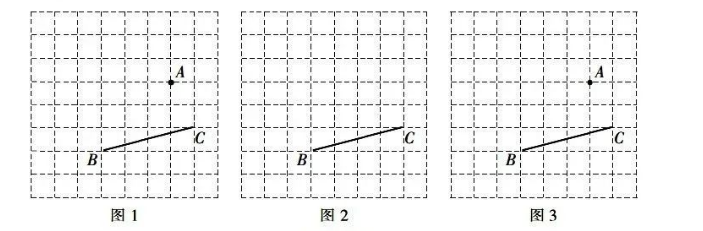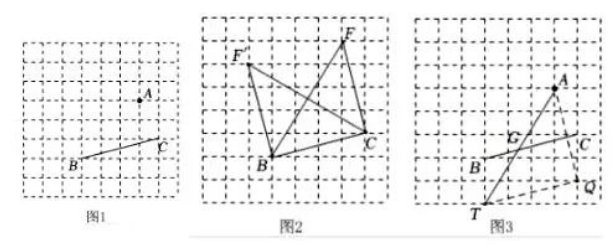(1) 在图 1 中, 在直线 $B C$ 的下方作格点 $D$ 使 $A D \perp B C$, 连结 $A D$, 垂足为 $H$.
(2) 在图 2 中找出所有可能的格点 $F$, 使 $\triangle B C F$ 是以 $B C$ 为直角边的等腰直角三角形, 并画出 $\triangle B C F$.
(3) 在图 3 中的线段 $B C$ 上画出点 $G$, 使 $\angle A G C=45^{\circ}$.【答案】